• ## 计算复杂性理论

千次阅读 2019-06-09 00:24:03
计算复杂性理论 在计算机算法中，计算复杂性是一个很重要的研究内容。计算复杂性理论（Computational complexity theory）被认为是理论计算机科学和数学的一个分支。 对于计算机而言，任何一个问题的求解都需要资源...
查看原文，点我
计算复杂性理论
在计算机算法中，计算复杂性是一个很重要的研究内容。计算复杂性理论（Computational complexity theory）被认为是理论计算机科学和数学的一个分支。
对于计算机而言，任何一个问题的求解都需要资源（即使是最简单的1+1的问题）。计算复杂性理论通过引入数学计算模型来研究这些问题以及定量计算解决问题所需的资源（时间和空间），从而将资源的确定方法正式化。
计算复杂性理论将计算问题按照在不同计算模型下所需时间资源的不同予以分类，就得到了常见的P、NP、NP完全、NP难这样的概念（note：P代表Polynomial，即多项式时间的概念）
P问题
一个问题如果在确定性图灵机上所需时间不会超过一个确定的多项式（以输入的长度为多项式的不定元），那么我们称这类问题的集合为P(polynomial time Turing machine)
通俗地来说P问题就是多项式时间内可解的问题
NP问题
可以在非确定型图灵机上在多项式时间内找出解的问题的集合
如果一个问题，可以在多项式时间内验证他的解是否正确，则该问题是一个NP问题。显然$P\in NP$ (note:到目前为止，P不等于NP)
NP-C问题(NP-Complete)
这里Complete是完备的意思
一个决定性问题C若是为NPC，则代表它对NP是完备的，这表示：

a) 该问题是一个NP问题
b) 所有属于NP的问题都可归约成该问题

对于一个NP-C问题，我们不可能尝试将所有的NP规约到它，所以通常采用下述方法证明一个问题是NP-C问题：

a) 证明给定该问题的一个解，可以在多项式时间验证该问题
b) 可以将一个已知的NP-C问题规约到该问题(已经证明的NPC问题：卡普的21个NPC问题或者A compendium of NP optimization problems)

在计算复杂度理论内，一个极度重要的成就是史提芬·古克在1971年证明出了第一个NP-完全问题— 布尔可满足性问题。

Cook定理(1971) 可满足问题属于NP-C

可满足问题（SAT）
可满足问题是判断任意给定的一个布尔表达式是否存在一个真赋值（如果有这样一个真赋值，则称该布尔表达式可满足）
NP-Hard
相较于NP-C问题，NP-Hard问题仅满足条件2

即所有的NP问题都可以规约到NP-Hard问题
通常通过将一个已知的NP-C问题规约到该问题来证明一个问题是NP-Hard问题

我们可以得到以下的关系图：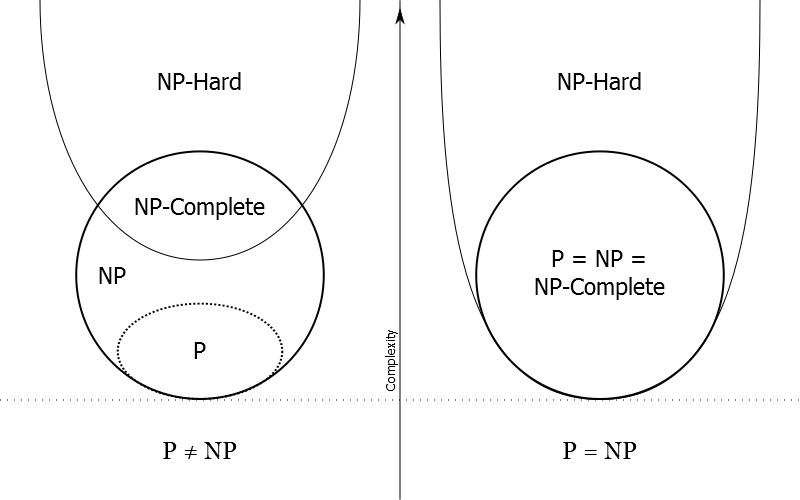证明$P=NP$是一个未解决的千禧年难题，当然如果最终证明$P=NP$，会发生很多“有趣”的事情：比如当下流行的密码理论将不再安全，可能这时能够期待的就只有量子加密技术的早日出现吧。
基本概念
多项式时间
多项式时间可解的问题，即P问题，通常被认为是一个易解的问题；一个多项式时间的算法往往也被认为是好的算法。然而多项式时间具体是什么含义？
在计算复杂度理论中，多项式时间（Polynomial time）指的是一个问题的计算时间m(n)不大于问题大小n的多项式倍数。用数学语言描述则为$m(n)= O(n^k)，k$为一常量值。
规约(reduction)
在可计算性理论与计算复杂性理论中，归约是将某个计算问题转换为另一个问题的过程。比较直观的说法：如果一个能有效解决问题B的算法，也可以作为解决问题A的子程序，则将问题A称为“可归约”到问题B，因此求解A并不会比求解B更困难。
规约是证明NP-Hard问题的一种常用方法，通常用$<=$这个符号来表示，如$p<=Q$，表示P is reducible to Q , or Q is the reduction from P or P is reduced to Q（P问题可以归约到Q问题，or可以把P归约到Q）。方便记忆的话，这里的规约符号可以记作小于且等于，即说明问题P至少比Q容易，或者Q至少比P难

问题A能够规约为问题B
a) 一个能求解问题B的算法一定可以用来求解问题A（以子问题的形式）
b) 求解问题A的难度一定不会比求解问题B的难度大（这里的难度大指的是求解过程需要更多的计算、存储资源等）。——>可以从侧面证明，如求解A的难度更大，而由于A可以规约为问题B，则可以用求解问题B的算法来求解问题A，则求解A的算法可以替换为一个难度更低的算法
规约具有传递性：A可以规约为B，B能规约为C，则A一定可以规约为C

可以说，一个问题归约为另一个问题的过程，是将问题复杂化的过程。归约得到问题的应用范围往往也扩大了。例如，一元二次方程的求解和一元一次方程的求解。
规约的类型较多，在本文中的规约特指多项式时间规约，即在多项式时间内将一个问题规约到另一个问题。
多项式归约主要做的就是以下两个转化（注意两个转化都要在polynomial的时间内完成）

把P的输入转化到Q的输入；
把Q的输出转化到P的输出。

3SAT
3SAT问题定义如下：

给定一个有穷的布尔变量集合$X=\{x_1,x_2,\cdots,x_n\},|X|=n$,每个变量取值为0或1，有一组子句（Clause）$C=\{C_1,C_2,\cdots,C_m\},|C|=m,C=C_1\cap C_2\cap\cdots \cap C_m$，每个$C_i$是由三个变量组成的析取范式，即$z_1\cup z_2 \cup z_3$.

其中

$x_i$是布尔变量（Variable）
一个布尔变量$x_i$或它的否定形式$\bar{x_i}$是文字（literal）
$C_i$为子句，一个子句包含3个文字（literal）

总的来说，一个SAT问题例子中包含一堆子句(Clause)，这一堆子句每个都包含3个文字(Literal),每个literal表示命题变元集中一个布尔变量（Variable）或它的否定形式
一个3SAT的例子：

$X=\{x_1,x_2,x_3\},C=\{C_1,C_2,C_3\},C_1=x_1\cup \bar{x_2}\cup x_3,C_2=\bar{x_1}\cup \bar{x_2}\cup x_3,C_3=\bar{x_1}\cup x_2\cup \bar{x_3}$
存在一个真值赋值：$x_1,x_2=1,x_3=1$，使得$C=1$，即该布尔表达式是可满足的

对于3SAT问题，有以下结论

$3SAT\in NPC$

0-1背包问题
众所周知，0-1背包问题是一个NP-C问题，应用动态规划算法可以得到伪多项式时间的算法，如何证明0-1背包问题是一个NP-C问题？
0-1 背包问题（0-1 Knapsack）在数学上的定义如下：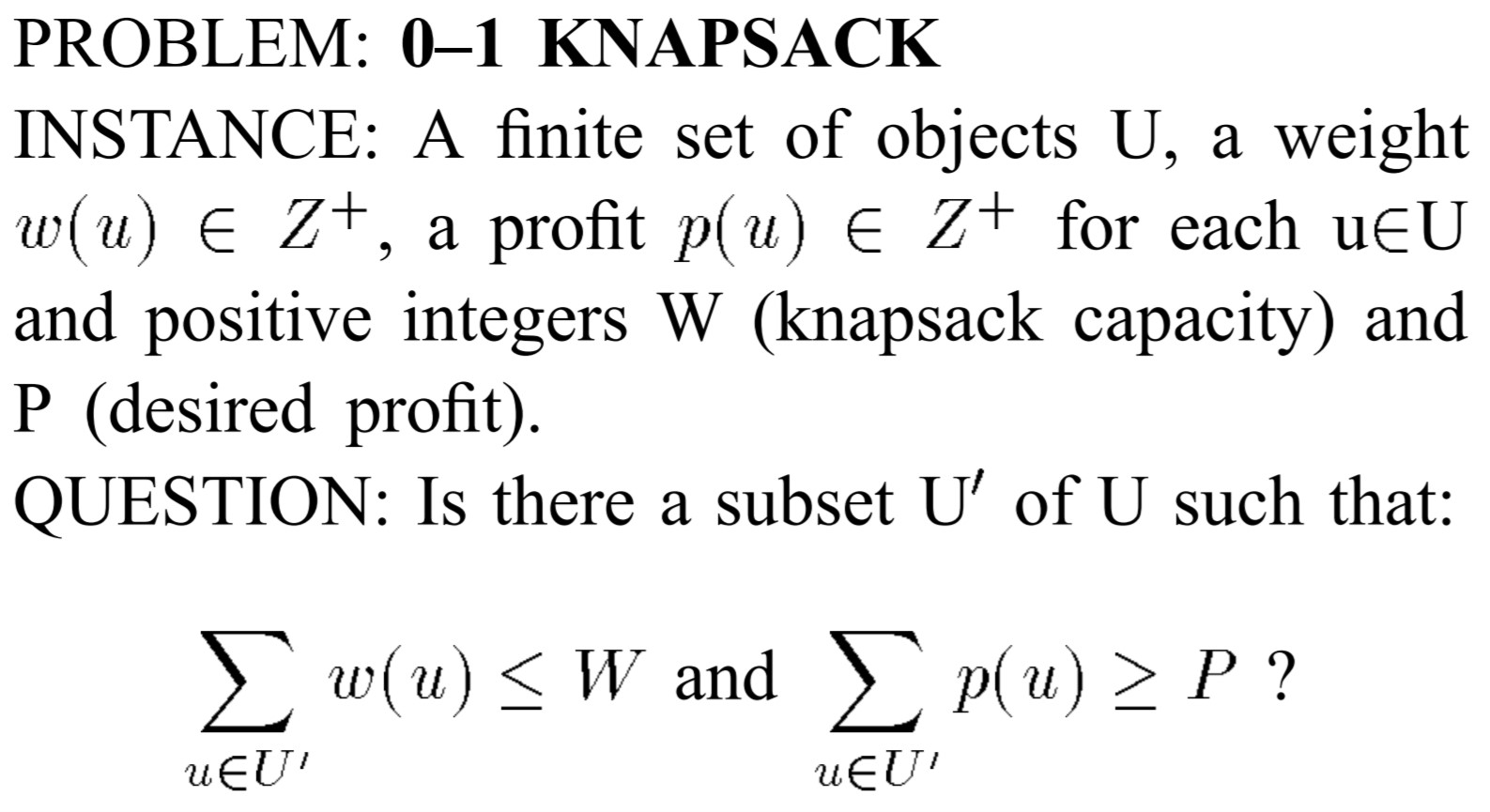通俗的来讲就是：

我们有n种物品，物品j的重量为$w_j$，价格为$p_j$,我们假定所有物品的重量和价格都是非负的。背包所能承受的最大重量为W.在限定的总重量内，我们如何选择，才能使得物品的总价格最高.如果限定每种物品只能选择0个或1个，则问题称为0-1背包问题

0-1背包问题本质上是一个优化问题，为了证明0-1背包问题是一个NP-C问题，我们首先引入0-1背包问题对应的判定问题（记作0-1 Knapsack Fill ）

我们有n种物品，物品j的重量为$w_j$，价格为$p_j$,我们假定所有物品的重量和价格都是非负的,限定每种物品只能选择0个或1个,是否存在一个选择策略，使得选择的物品总重量为W

看上去似乎引入0-1 Knapsack Fill问题没有任何用处，实际上对于优化问题和其对应的判定问题，有如下结论：

一个优化问题，不会比其对应的判定问题简单

由此，可以从0-1 Knapsack Fill问题入手，来证明0-1 Knapsack问题的复杂性

证明0-1 Knapsack Fill $\in$ P问题
显然给定0-1 Knapsack的一个解,可以在多项式时间内验证该解是否正确：对选取的物品集合做一次遍历,累加得到价格和重量总和，即可验证。所以0-1 Knapsack Fill $\in$ P问题.

将一个已知的NP-C问题规约到0-1 Knapsack Fill问题(多项式时间内)
证明3SAT<=0-1 Knapsack Fill

对于一个3SAT问题，设$X=\{x_1,x_2,\cdots,x_n\},|X|=n$，有一组子句$C=\{C_1,C_2,\cdots,C_m\},|C|=m,C=C_1\cap C_2\cap\cdots \cap C_m$。
对于0-1 Knapsack Fill问题，存在一组物品$U$和背包容量W，我们希望得到结论：当且仅当C可满足时，存在物品集合的子集$U^{'}\in U$ 使得$\sum_{u\in U^{'}} w(u)=W$。

为了将3SAT规约到0-1 Knapsack Fill，做出以下定义：

对于每个布尔变量$x_i$，对应$u_i和\bar{u_i}$
对于每个子句$c_j$，定义两个补偿对象（compensating objects）$co1_j和co2_j$
用$(n+m)$长度的3进制数表示物品重量$w(u)$，其中第$i$个数字与布尔变量$x_i$相关；第$(n+j)$个数字，与子句$c_j$相关。

对于$u_i和\bar{u_i}$：

最右边$n$个数字:第$i$个数字为1，其余数字为0
最左边$m$个数字:如果$x_i(与u_i$相关)或者$\bar{x_i}(与\bar{u_i}相关)$在子句$c_j$中出现，则第$n+j$个数字为1，其余数字为0

对于$co1_j和co2_j$：

最右边$n$个数字:全为0
最左边$m$个数字（用来标识对应的子句$c_j$）:第$n+j$个数字为1，其余数字为；$co1_j和co2_j$的重量相同

背包容量$W$

最右边$n$个数字:全为1
最左边$m$个数字:全为3

通过上述转化过程，我们将一个3SAT问题归约到了0-1 Knapsack Fill实例（显然该过程是在多项式时间内，因为每一步的转化过程都是确定的），该实例有以下特点：

$U=\{u_i,\bar{u_i}: 1\leq i\leq n\}\cup\{co1_j,co2_j: 1\leq j \leq m\}$

$w(u_i)由(n+m)$个二进制数组成

第$i$个数字为1
当且仅当$x_i$在子句$c_j$中出现时，第$n+j$个数字为1
其余所有数字均为0

$w(\bar{u_i})由(n+m)$个二进制数组成

第$i$个数字为1
当且仅当$\bar{x_i}$在子句$c_j$中出现时，第$n+j$个数字为1
其余所有数字均为0

$w(co1_j)=w(co2_j)由(n+m)$个二进制数组成

第$n+j$个数字为1
其余所有数字均为0

背包容量$W$为$(n+m)$长度的二进制数

最右的$n$个数为1
最左的$m$个数为3

对于任意一个3SAT问题，我们都可以通过上述过程转化为一个0-1 Knapsack Fill问题，为证明0-1 Knapsack Fill问题的复杂性，只需证明：当且仅当C可满足时，存在物品集合的子集$U^{'}\in U$ 使得$\sum_{u\in U^{'}} w(u)=W$

先证明存在物品集合$U^{'}\in U$使得$\sum_{u\in U^{'}} w(u)=W$时，C可满足

假设已得到部分物品集合$U^{'}\in U$使得$\sum_{u\in U^{'}} w(u)=W$
最右边的$n$个数字全为1：保证$u_i,\bar{u_i}$有且仅有一个出现在集合$U^{'}$(否则第$i$个数字的值为0或2，与假设冲突)——>可以得到一组3SAT中布尔变量的赋值，记作$v$。

如果$u_i\in U^{'}$,则有$x_i^{v}=1$
如果$\bar{u_i}\in U^{'}$,则有$\bar{x_i}^{v}=1或者x_i^v=0$

最右边的$m$个数字均为3：保证每个子句$c_j$均是可满足的，即$C$可满足

如果$co1_j,coj_2均不属于U^{'}$，有$\sum_{u\in U^{'},u=u_i or u=\bar{u_i}} w(u)=3$。所以子句$c_j$中的布尔变量均为1，所以每个子句均可满足
有且仅有$co1_j,coj_2中的一个属于U^{'}$，，有$\sum_{u\in U^{'},u=u_i or u=\bar{u_i}} w(u)=2$。所以子句$c_j$中有两个布尔变量为1，所以每个子句均可满足（合取范式）
$co1_j,coj_2均属于U^{'}$，，有$\sum_{u\in U^{'},u=u_i or u=\bar{u_i}} w(u)=1$。所以子句$c_j$中有一个布尔变量为1，所以每个子句均可满足（合取范式）

证明当C可满足时，存在物品集合的子集$U^{'}\in U$ 使得$\sum_{u\in U^{'}} w(u)=W$

与上述证明过程类似，这里不再赘述

通过上述过程，我们有3SAT<=0-1 Knapsack Fill

输入过程：$x_i,\bar{x_i}与u_i,\bar{u_i}$对应；$x_i,\bar{x_i}是否在子句c_j中出现由第(n+j)个数字标识$，结合而成的$(n+m)$个数字表示物品重量
输出过程：当且仅当C可满足时，存在物品集合的子集$U^{'}\in U$ 使得$\sum_{u\in U^{'}} w(u)=W$。

给出一个3SAT<=0-1 Knapsack Fill的例子：

3SAT:
$X=\{x_1,x_2,x_3,x_4,x_5\},C=\{c_1,c_2,c_3,c_4\},c_1=\{x_1,\bar{x_2},x_4\},c_2=\{x_2,\bar{x_3},\bar{x_5}\},c_3=\{x_3,x_4,x_5\},c_4=\{\bar{x_1},x_2,\bar{x_5}\}$
对应的0-1 Knapsack Fill问题：
$U=\{u_1,\bar{u_1},u_2,\bar{u_2},u_3,\bar{u_3},u_4,\bar{u_4},u_5,\bar{u_5},co1_1,co2_1,co1_2,co2_2,co1_3,co2_3,co1_4,co2_4\}$,W=333311111.
物品集合U中物品对应重量为：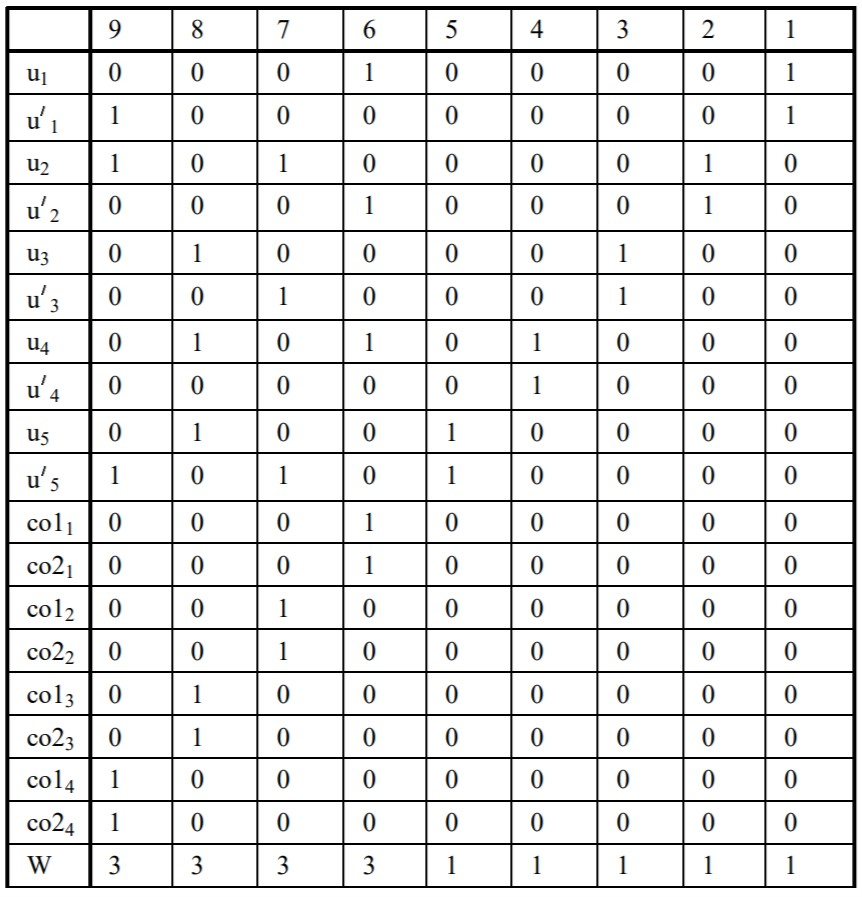综合证明1和2，可知0-1 Knapsack Fill$\in$ NPC
对于0-1 knapsack问题，给定其一个解，无法在多项式时间内验证该解是否正确：

可以从侧面给出不严谨证明：0-1 knapsack是一个优化问题，如果能在多项式时间内验证一个解，相当于能在多项式时间内求出该优化问题的最优解

综上：可知0-1 knapsack$\in$NP-Hard


展开全文• 计算复杂性理论（Computational complexity theory）是理论计算机科学和数学的一个分支，它致力于将可计算问题根据它们本身的复杂性分类，以及将这些类别联系起来。一个可计算问题被认为是一个原则上可以用计算机...
       计算复杂性理论（Computational complexity theory）是理论计算机科学和数学的一个分支，它致力于将可计算问题根据它们本身的复杂性分类，以及将这些类别联系起来。一个可计算问题被认为是一个原则上可以用计算机解决的问题，亦即这个问题可以用一系列机械的数学步骤解决，例如算法。

如果一个问题的求解需要相当多的资源（无论用什么算法），则被认为是难解的。计算复杂性理论通过引入数学计算模型来研究这些问题以及定量计算解决问题所需的资源（时间和空间），从而将资源的确定方法正式化了。其他复杂性测度同样被运用，比如通信量（应用于通信复杂性），电路中门的数量（应用于电路复杂性）以及中央处理器的数量（应用于并行计算）。计算复杂性理论的一个作用就是确定一个能或不能被计算机求解的问题的所具有的实际限制。

在理论计算机科学领域，与此相关的概念有算法分析和可计算性理论。两者之间一个关键的区别是前者致力于分析用一个确定的算法来求解一个问题所需的资源量，而后者则是在更广泛意义上研究用所有可能的算法来解决相同问题。更精确地说，它尝试将问题分成能或不能在现有的适当受限的资源条件下解决这两类。相应地，在现有资源条件下的限制正是区分计算复杂性理论和可计算性理论的一个重要指标：后者关心的是何种问题原则上可以用算法解决。

展开全文算法
• 量子计算复杂性理论综述，详细介绍了，量子计算复杂性理论相关研究大数据
• 关于计算复杂性理论相关知识的pdf文档，介绍了计算复杂性理论的发展历程及其相关技术
• 算法分析与 计算复杂性理论;课程简介;课程内容;预计进度安排;教材与参考书;学习安排;引言: 理论上的可计算与现实上的可计算 ;投资问题;蛮力算法的代价; T(n) = 2 T(n?1) + 1T(1) = 1;其他问题;Algorithm + Data ...
• 计算复杂性理论参考资料（英文，169页）：Computation Complexity, by Laszlo Lovasz
• （1）－ 可计算性理论与计算复杂性理论 已有 1843 次阅读 2015-6-6 00:07 |个人分类:不确定性问题和算法讨论|系统分类:科研笔记|关键词:P versus NP 计算复杂性理论 可计算性理论 我们一直在解读，“P...


什么是“判定问题”？（1）－ 可计算性理论与计算复杂性理论

已有 1843 次阅读 2015-6-6 00:07 |个人分类:不确定性问题和算法讨论|系统分类:科研笔记|关键词:P
versus NP 计算复杂性理论 可计算性理论

我们一直在解读，“P versus NP”之所以成为“世纪难题”，失足于NP定义：NP＝Nondeterministic Polynomial time（不确定性多项式时间），遂有流行观念“NP是多项式时间可验证的”，与此相关，还有一个流行观念“NP是可计算的”。这些观念直接导致计算复杂性理论（Computational complexity theory）与可计算性理论（Computability theory）相分离，作为计算复杂性理论基础的NP完备理论（NP－completeness theory），就成了无源之水，无本之木！

于可计算性理论，按人们一般的理解，顾名思义是研究“可计算性”，即研究哪些问题是可计算的，然而，若追本溯源，可以看到，此理论的目的是通过“可计算性”，研究与之相对的“不可计算性”。“可计算性”概念源于古老的西方哲学问题：思维机械化，其中心议题是设想通过将思维表达成计算，来检验和消除认知错误，正如莱布尼茨所说：

－ 纠正我们推理的唯一方式是使它们同数学一样准确、明晰，这样我们能一眼看出我们的错误，并且在人们有争执的时候，可以简单的说，让我们计算「calculemus」，而无须进一步的忙乱，就能看出谁是正确的。（The only way to rectify our reasonings is to make them as tangible as those of the Mathematicians, so that we can find our error at a glance, and when there
are disputes among persons, we can simply say: Let us calculate [calculemus], without further ado, to see who is right.）

于20世纪初，希尔伯特正式提出了利用计算机彻底系统解决所有数学问题的计划，其中的希尔伯特第10问题，又称“丢番图方程问题”，即探究求解任意的丢番图方程的算法的存在性。然而，哥德尔几乎在同时提出了“哥德尔不完备定理”，证明了不存在着这样的算法，它能正确回答有关初等数论的全部问题。接着，图灵进一步提出普遍意义上的计算机模型－“图灵机”，严格证明了不存在这样的图灵机，一般性地表达为“判定问题（Decision Problem）”，由此形成了可计算性理论的核心内容。

于20世纪40年代，发明了基于冯·诺伊曼结构的实际计算机，计算机应用广泛开展起来，于实际应用中出现了一些计算困难的问题，“计算复杂性理论”于此创生。一般说来，计算复杂性理论研究“复杂性”，即研究那些计算困难问题的“复杂性”，库克（Cook）于1971年的那篇论文《The Complexity of Theorem Proving Procedures》，引入“Nondeterministic Turing machine（NDTM）”，将那些计算困难的问题定义为NP（Nondeterministic Polynomial
time），由于NDTM的本质是图灵机（TM），遂有诸如“NP是多项式时间可验证的”、“NP是可计算的”的流行观念，其相关理论称之“NP完备理论”。

由此可见，原本NP的提出是针对计算困难的问题，与P相对，然而NP的流行观念混淆了NP与P的本质区别，导致NP的本质“不确定性”消失，是有计算复杂性理论与可计算性理论完全脱节，这是造成现有的NP完备理论严重困难的最根本性的原因。


展开全文• 线性规划 AGH大学“运筹学和计算复杂性理论”课程的生产者和最佳生产选择程序JavaScript
• 数学基础数学基础 符符号说说明 取整函数 对数 阶乘 求和 估计和式的上界估计和式的上界 递推方程求解 递推方程中涉及递推方程中涉及 xx和和 xx 的处理方法的处理方法 1 符号说明符号说明 取整函数取整函数 x 小于...
• 概念与语言 确定自动机，适合学过编译原理的高年级同学和研究生。
• 判定问题关注同样的结构、同样的指标，但是不同于优化问题的是，它不在关注指标的最大、最小值，而是引进一个参数k，并问一个“是与否”的问题 研究判定问题的意义是什么呢： 1、能为研究问题的复杂性发挥什么作用？...
一、归约的意义

求解一个算法问题的时候，我们往往可以直观地感受到有些问题是比较难的，有些问题是比较简单的，但是我们并不能因为没有设计出一个比较高效的算法，就说它是一个难问题，所以问题的难易是相对的，我们需要一个科学的手段来界定问题的难易

我们可以用问题之间的归约，来界定两个问题之间相对难易程度的基本手段

二、优化问题与判定问题

很多经典的难问题都是优化问题，而一个优化问题往往可以转换成对应的判定问题。

一般而言，优化问题是关注某种特殊的结构，并希望优化该结构的某种指标

最大团问题就是典型的优化问题、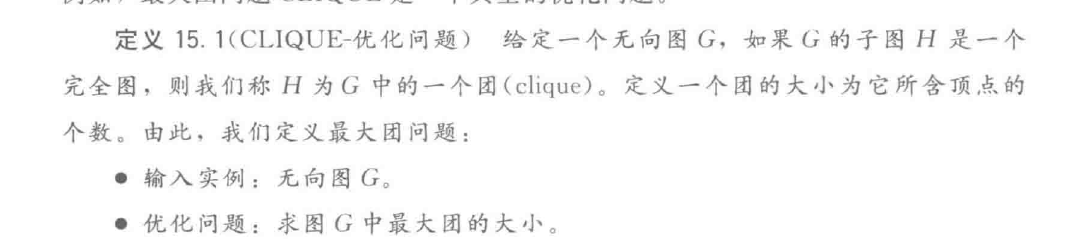一个优化问题往往可以定义其对应的判定问题。判定问题关注同样的结构、同样的指标，但是不同于优化问题的是，它不在关注指标的最大、最小值，而是引进一个参数k，并问一个“是与否”的问题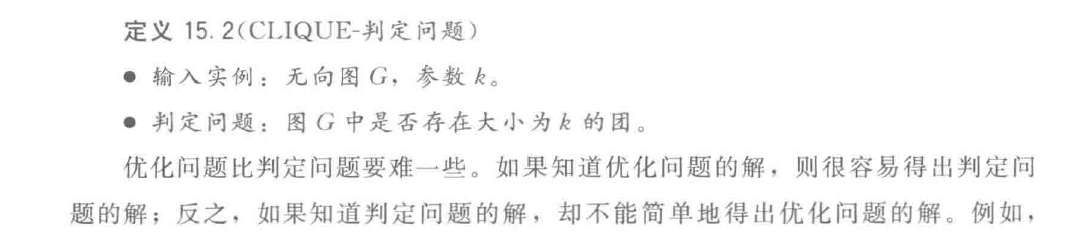研究判定问题的意义是什么呢：

1、能为研究问题的复杂性发挥什么作用？

2、判定问题比优化问题更简单，那么研究判定问题能否全面反映该问题的复杂性

三、归约的定义

问题P可归约到问题Q：问题P可以间接地通过解决问题Q来实现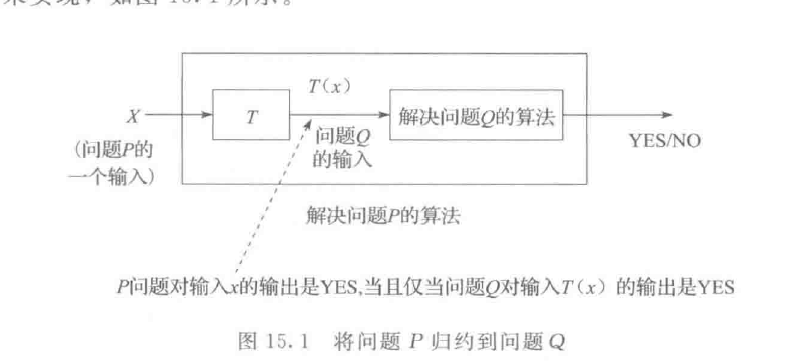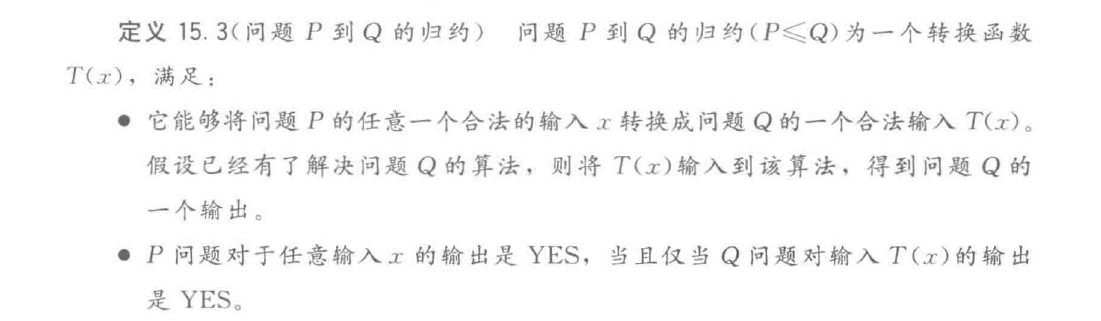四、多项式时间

我们将多项式时间可解的问题称作P问题

对问题这样分类的意义？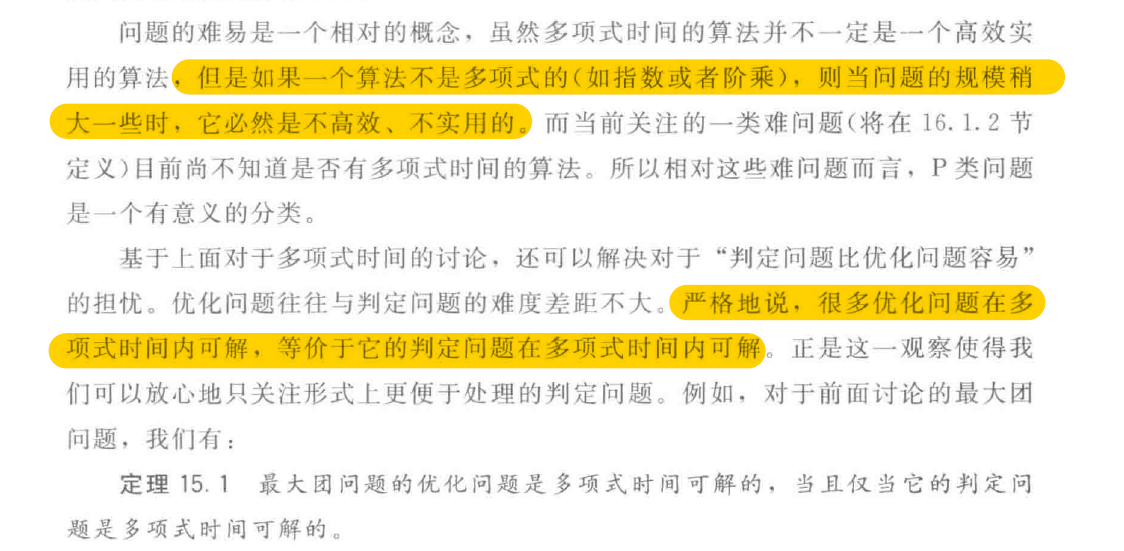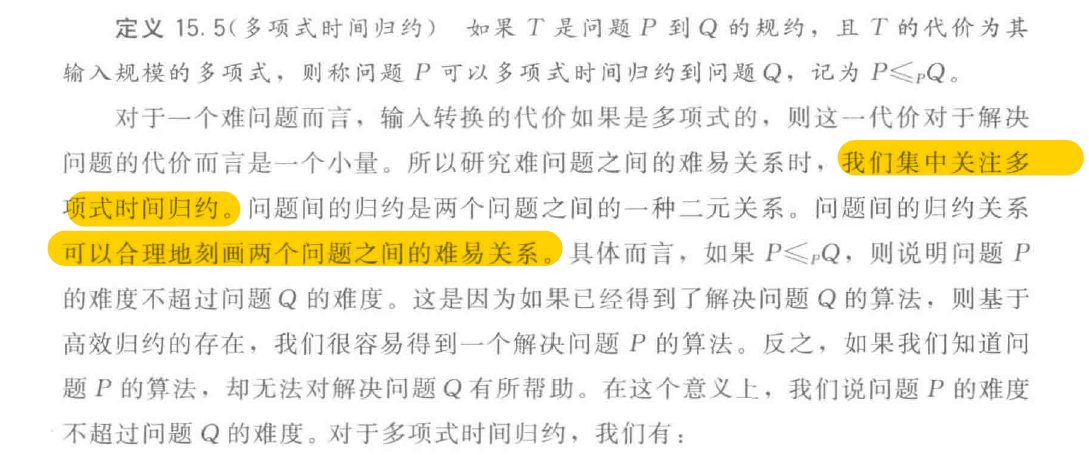展开全文• 什么是NP系列问题？今天来看看这些问题。...我们主要学习的内容是算法分析与问题的计算复杂性，以及分治策略、动态规划、贪心算法、回溯与分支限界等的学习。 学习是漫长的，期待后面将算法知识学好。算法 NP难 NP-hard
• 能不能有效计算的临界问题 问题简述： 如下红色路线就是本题的解 对货郎问题进行建模 从城市C1到C2的距离加上从Ck2到Ck3的距离，一直加到Ck(n-1)到达Cnk城市的距离,再加上从第n个城市回到第n个城市的距离。 这...算法 动态规划 深度学习 数据挖掘
• 博客中思维导图的高清PDF版本,可关注公众号 一起学计算机 点击 资源获取 获得 目录 1.2算法设计两个例子:调度问题和投资问题 1.3问题计算复杂度的界定:排序问题 ​1.4货郎问题与计算复杂性理论 ​1.5算法及其时间...
• 分享计算机研究生课程资料 主要内容 1 Turing机 2 计算复杂性理论 3 NP完全性理论的基本概念 4 NP完全性证明 5 用NP完全性理论分析问题 6 NP难度
• 计算复杂性理论是用数学方法研究使用数位计算机解决各种算法问题困难度的理论。《计算复杂性导论》对计算机科学中这一重要理论做了全面的介绍。其内容包含基本理论，如计算模型NP-完全性，以及较深入的课题，如线路...NP完全问题
• 一、计算理论内容概览、 二、计算问题的判定性、 三、计算问题的 有效性、 四、时间复杂性度量、 五、算法有效性 数学定义需求、 六、输入表示、 七、时间复杂度时间复杂度 图灵机
• ## 计算复杂性综述

千次阅读 2019-05-15 15:50:10
文章目录计算复杂性前言正文一、计算复杂性理论基本问题（一）时间复杂度（二）Cook-Karp论题（三）Church论题二、判定问题类（一）P问题(Polynomial Problem)（二）NP问题(Non-deterministic Polynomial Problem)...
• 中国科学 信息科学 年 第 卷 第 期 SCIENTIA SINICA Informationis 信息科学与技术若干前沿问题评述专刊 大数据计算复杂性理论与算法研究进展 * 李建中 李英姝 哈尔滨工业大学计算机学院, 哈尔滨 150001 ...
• 计算复杂性理论是用数学方法研究使用数位计算机解决各种算法问题困难度的理论。《计算复杂性导论》对计算机科学中这一重要理论做了全面的介绍。其内容包含基本理论，如计算模型NP-完全性，以及较深入的课题，如线路...
• 自动机理论、语言和计算导论课后习题答案doc版，包含2到9章部分重要习题答案； 计算复杂性导论pdf版。
• 本书是一本全面阐述计算机复杂性理论及其近年来进展的教科书，主要包含算法图灵机、可计算性等有关计算复杂理论的基本概念；布尔逻辑、一阶逻辑、逻辑中的不可判定性等复杂性理论的基础知识；P与NP、NP完全等各复杂...
• 本书是一本全面阐述计算机复杂性理论及其近年来进展的教科书，主要包含算法图灵机、可计算性等有关计算复杂理论的基本概念；布尔逻辑、一阶逻辑、逻辑中的不可判定性等复杂性理论的基础知识；P与NP、NP完全等各复杂...
• 内容提要: 本书论述了形式语言的基本内容，包括有限自动机、下推机和图灵机的基础理论，讨论了如分治策略、动态规划、回溯法、贪心法以及概率算法的基本技术；同时，也给出了计算复杂性理论的基本知识。
• 计算复杂性理论的研究是计算机科学最重要的研究领域之一，而Christos H．Papadmitriou是该领域最著名的专家之一。本书是一本全面阐述计算复杂性理论及其近年来进展的教科书，主要包含算法图灵机、可计算性等有关计算......